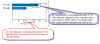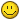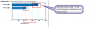## Recommended Posts

My requirement is to make y-axis value show integer in Bar model ,but it will convert to strange chart, if I set yAxisValueDecimals=2, it will show rightly.

My question:

How fushionchart to format decimal to integer, does any suggestion to fix this problem.

BTW:

Fushionchart verison:FusionCharts_Enterprise_v3_2

My data xml:

```<chart animation="0" baseFont="Arial" baseFontColor="000000"  baseFontSize="9" bgColor="FFFFFF" borderColor="FFFFFF"  canvasRightMargin="10" chartBottomMargin="20" chartLeftMargin="20"  chartRightMargin="20" chartTopMargin="20" decimals="2"  defaultNumberScale="" maxLabelWidthPercent="20"  numberScaleUnit="KB,MB,GB" numberScaleValue="1024,1024,1024" palette="3"  placeValuesInside="1" plotGradientColor="" setAdaptiveYMin="0"  showBorder="1" showShadow="0" showValues="1" yAxisName="Message size"  yAxisValueDecimals="0">
<set color="0066AA" label="[email protected]" tooltext="[email protected]  3.04KB" value="3110"/>
<set color="1D7BBF" label="[email protected]" tooltext="[email protected]  1.66KB" value="1700"/>
<set label=" "/>
<set label=" "/>
<set label=" "/>
</chart>```

details chart and comments, see attachments.Edited by TomWebsense

##### Share on other sitesHi,

Please find the modified XML below:

<chart animation="0" baseFont="Arial" baseFontColor="000000" baseFontSize="9" bgColor="FFFFFF" borderColor="FFFFFF" canvasRightMargin="10" chartBottomMargin="20" chartLeftMargin="20" chartRightMargin="20" chartTopMargin="20" maxLabelWidthPercent="20" numberScaleUnit="KB,MB,GB" numberScaleValue="1024,1024,1024" palette="3" placeValuesInside="1" plotGradientColor="" setAdaptiveYMin="0" showBorder="1" showShadow="0" showValues="1" yAxisName="Message size" yAxisValueDecimals="0" formatNumber='1' decimals='0' >

<set color="0066AA" label="[email protected]" tooltext="[email protected] 3.04KB" value="3110"/>

<set color="1D7BBF" label="[email protected]" tooltext="[email protected] 1.66KB" value="1700"/>

<set label=" "/>

<set label=" "/>

<set label=" "/>

</chart>

Hope this helps.##### Share on other sites

Thanks for suggestions!

After I try your providing xml, it still has some problems:

1. 2KB in x-axis label value has double.

2. There still has gap between dataplot value and vertical line.

Whether currently fushionchart could not make it show rightly?

Any suggestions?

BTW: could you tell me what is the rule for fushionchart is to apply as converting decimal to integer?

Thanks!

Tom:)Edited by TomWebsense